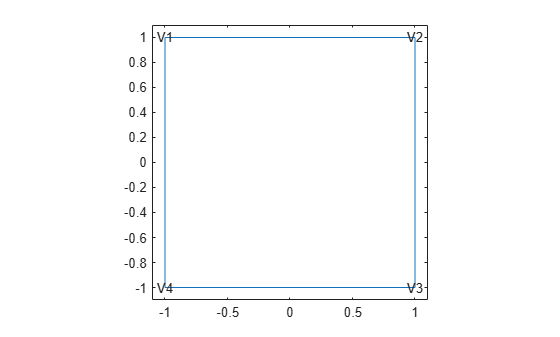# vertexIC

Initial conditions on geometry vertex

Since R2023a

## Description

A `vertexIC` object specifies the type of initial condition on a vertex of a geometry. An `femodel` object contains an array of `vertexIC` objects in its `VertexIC` property.

For separate assignments to a geometric region (or the entire geometry) and the boundaries of that region, the solver uses the specified assignment on the region and chooses the assignment on the boundary as follows. The solver gives an `EdgeIC` assignment precedence over a `FaceIC` assignment, even if you specify a `FaceIC` assignment after an `EdgeIC` assignment. The assignments in order of precedence are `VertexIC` (highest precedence), `EdgeIC`, `FaceIC`, and `CellIC` (lowest precedence).

## Creation

### Syntax

``model.VertexIC(VertexID) = vertexIC(Name=Value)``
``model.VertexIC = vertexIC(Name=Value)``

### Description

example

````model.VertexIC(VertexID) = vertexIC(Name=Value)` creates a `vertexIC` object and sets properties using one or more name-value arguments. This syntax assigns the specified structural or thermal initial condition to the specified vertices of the geometry stored in the `femodel` object `model`. For example, `model.VertexIC([1 2]) = vertexIC(Temperature=25)` specifies the temperature on vertices 1 and 2.```

example

````model.VertexIC = vertexIC(Name=Value)` assigns the specified initial condition to all vertices of the geometry. For example, `model.VertexIC = vertexIC(Temperature=25)` specifies the initial temperature on all vertices.model.VertexIC = vertexIC(Temperature = 25)```

### Input Arguments

expand all

Vertex IDs, specified as a vector of positive integers. Find the vertex IDs using `pdegplot` with the `VertexLabels` value set to `"on"`.

Data Types: `double`

## Properties

expand all

Initial displacement, specified as a numeric vector or function handle. A numeric vector must contain two elements for a 2-D model and three elements for a 3-D model. The elements represent the components of initial displacement.

Use a function handle to specify spatially varying initial displacement. The function must return a two-row matrix for a 2-D model and a three-row matrix for a 3-D model. Each column of the matrix corresponds to the initial displacement at the coordinates provided by the solver. For details, see Nonconstant Parameters of Finite Element Model.

Initial velocity, specified as a numeric vector or function handle. A numeric vector must contain two elements for a 2-D model and three elements for a 3-D model. The elements represent the components of initial velocity.

Use a function handle to specify spatially varying initial velocity. The function must return a two-row matrix for a 2-D model and a three-row matrix for a 3-D model. Each column of the matrix corresponds to the initial velocity at the coordinates provided by the solver. For details, see Nonconstant Parameters of Finite Element Model.

Initial temperature or initial guess for temperature, specified as a real number or function handle. Use a function handle to specify spatially varying initial temperature. For details, see Nonconstant Parameters of Finite Element Model.

## Examples

collapse all

Specify initial displacement for an `femodel` object representing a transient structural problem.

Create an `femodel` object for solving a transient structural problem, and assign the unit square geometry to the model.

```model = femodel(AnalysisType="structuralTransient", ... Geometry=@squareg);```

Plot the geometry with the vertex labels.

```pdegplot(model.Geometry,VertexLabels="on"); xlim([-1.1 1.1]) ylim([-1.1 1.1])```The default initial displacement on the entire geometry is zero. Specify a small nonzero initial displacement on vertex 1.

```model.VertexIC(1) = vertexIC(Displacement=[0 10^(-5)]); model.VertexIC```
```ans = 1x4 vertexIC array Properties for analysis type: structuralTransient Index Displacement Velocity Temperature 1 [0 1.0000e-05] [] [] 2 [] [] [] 3 [] [] [] 4 [] [] [] Show all properties ```

Specify initial temperature for an `femodel` object representing a transient thermal problem.

Create an `femodel` object for solving a transient thermal problem, and assign the unit square geometry to the model.

```model = femodel(AnalysisType="thermalTransient", ... Geometry=@squareg);```

Plot the geometry with the vertex labels.

```pdegplot(model.Geometry,VertexLabels="on"); xlim([-1.1 1.1]) ylim([-1.1 1.1])```Because there is no default initial temperature, you must specify the initial temperature for the entire geometry.

```model.FaceIC = faceIC(Temperature=0); model.FaceIC```
```ans = 1x1 faceIC array Properties for analysis type: thermalTransient Index Temperature 1 0 Show all properties ```

Specify a small nonzero initial temperature on all corners of the square.

```model.VertexIC = vertexIC(Temperature=0.1); model.VertexIC```
```ans = 1x4 vertexIC array Properties for analysis type: thermalTransient Index Temperature 1 0.1000 2 0.1000 3 0.1000 4 0.1000 Show all properties ```

## Version History

Introduced in R2023a